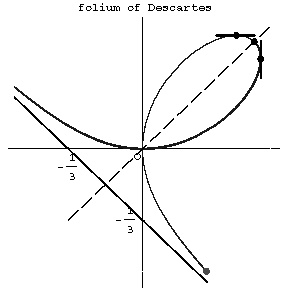# Folium of Descartes

A plane algebraic curve of order three which is given in Cartesian coordinates by the equation; the parametric equations are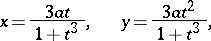whereis the tangent of the angle between the radius vector of the curve and the-axis. The folium of Descartes is symmetric about the axis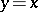(see Fig.). The tangent lines are parallel to the coordinate axes at the points with coordinates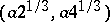and. The coordinate origin is a nodal point with the coordinate axes as tangent lines. The asymptote is given by. The surface area enclosed between the curve and the asymptote is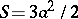. The surface area of the loop is. Named after R. Descartes who was the first to study it in 1638.